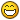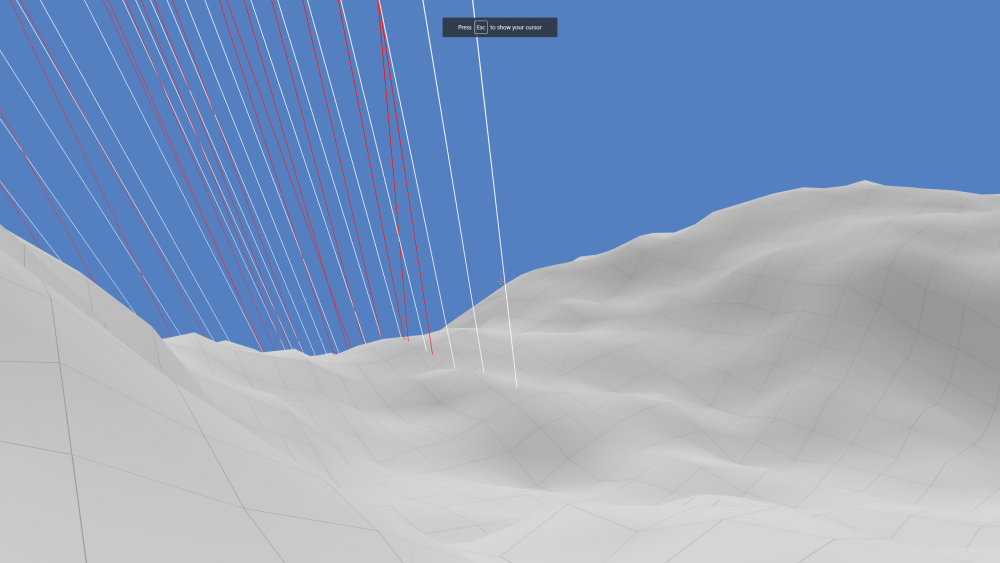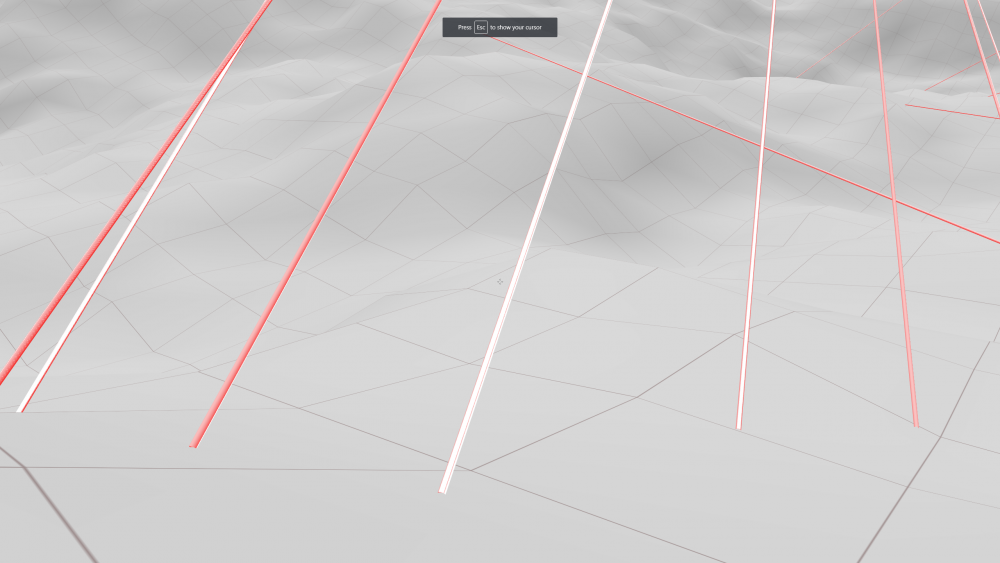# solved Math for moving rotated objects

## Recommended Posts

What are the ways of moving a rotated object in the direction that it is facing (or left, or right, or backwards, up, or down)? How much does this change if the game is a first person shooter (player's head always points up to Y) versus a space ship game (where the spaceship can go upside down and even barrel roll) ?

I understand that I can take a vector such as BABYLON.Vector3.Up() and locallyTranslate it via a mesh that has a rotation... but how do I describe this direction as a Vector3 (instead of immediately applying it to the mesh) so that I can use moveWithCollisions(movementVector)?

How hard is it to do this math manually?

Should I be studying quaternions?

ThanksHere's some of my movement code (which works, ish) but is very indirect:

``````// face player mesh to face the same direction as the camera
this.mesh.lookAt(
new BABYLON.Vector3(
-command.rotationX,
-command.rotationY,
-command.rotationZ
)
)
)

// controls: forward, backward, left, right
let unit = BABYLON.Vector3.Zero()
if (command.forward) { unit.z += 1 }
if (command.backward) { unit.z -= 1 }
if (command.left) { unit.x -= 1 }
if (command.right) { unit.x += 1 }
unit.normalize() // to prevent diagonal movement being faster

// full vector, movement and magnitude
let velocityCoef = this.speed * command.delta
this.velocity.x += unit.x * velocityCoef
this.velocity.y += unit.y * velocityCoef
this.velocity.z += unit.z * velocityCoef

// no idea how to align the velocity vector with
// the direction we're facing... so locallyTranslate it
let temp = this.mesh.position.clone()
this.mesh.locallyTranslate(this.velocity)
// but locallyTranslate doesnt do collisions.. so lets just
// teleport back to where we were and calculate what vector
// we just moved along
let diff = this.mesh.position.subtract(temp)
this.mesh.position.copyFrom(temp)
// now we have the vector that we would've moved, let's
// try it again with collisions
this.mesh.moveWithCollisions(diff)

this.velocity.x = 0
this.velocity.y = 0
this.velocity.z = 0``````

##### Share on other sites

What does one pass to getDirection?

##### Share on other sites

Iv'e been experimenting with a few things, mostly centered around TransformCoordinates, and Matrix.RotationAxis(axis, mesh.rotation).

I've been trying this for forward on a mesh:

``````let forward = BABYLON.Vector3.TransformCoordinates(BABYLON.Vector3.Forward(), mesh.getWorldMatrix())
let dir = forward.subtract(mesh.position).normalize()``````

Then this for forward on a camera:

``let cameraRay = camera.getForwardRay().direction``

And then for multiplayer, when moving a player on the server, I send the cameraRay and the controls over the network and move forward/back and strafe left/right like this:

``````let camVector = new BABYLON.Vector3(
command.cameraVectorX,
command.cameraVectorY,
command.cameraVectorZ
)

// rotates the player

let unit = BABYLON.Vector3.Zero()
if (command.forward) { unit.z += 1 }
if (command.backward) { unit.z -= 1 }
if (command.left) { unit.x -= 1 }
if (command.right) { unit.x += 1 }
unit.normalize()

let matrix = BABYLON.Matrix.RotationAxis(BABYLON.Axis.Y, this.mesh.rotation.y)
unit.x * this.speed * command.delta,
unit.y * this.speed * command.delta,
unit.z * this.speed * command.delta,
)

let movement = heading.clone() // no need to clone
if (command.jump) {
// no-accel jetpack
movement.y += 10 * command.delta
} else {
// really fake no-accel gravity
movement.y -= 10 * command.delta
}

let movementVector = BABYLON.Vector3.TransformCoordinates(movement, matrix)
this.mesh.moveWithCollisions(movementVector)

// and then for shooting, ``````

^ `command` is the network object holding the data from the client

It is getting a little better, still not sure if these are good ways to do things or not

##### Share on other siteslooks great to me##### Share on other sites

I've added firing a Ray forward to the above logic and I'm running into a problem. I begin calculating the direction of the ray similarly to the forward-ish logic from above:

``````let f = BABYLON.Vector3.TransformCoordinates(BABYLON.Vector3.Forward(), mesh.getWorldMatrix())
console.log('f', f)
console.log('mesh.position', this.mesh.position)
console.log('mesh.rotation', this.mesh.rotation)``````

Problem: `f` is different on server and client, even though mesh.position and mesh.rotation are the same. So I guess mesh.getWorldMatrix() is based off more than just position and rotation..is that correct? What other data do I need to synchronize? I think some part of the transform is out of sync.

Log output from server:

`````` f t {
   x: 87.71782332658768,
   y: 28.766663193702698,
   z: 39.23026758432388 }
 mesh.position t {
   x: 87.25551778257102,
   y: 29.250780211047058,
   z: 38.58501679257934 }
 mesh.rotation t { x: 0.6739999993520329, y: 0.7990000362848866, z: 0 }``````

Log output from client:

``````f t {x: 87.81546431779861, y: 28.62666380405426, z: 39.12993723154068}
mesh.position t {x: 87.25551778257102, y: 29.250780211047058, z: 38.58501679257934}
mesh.rotation t {x: 0.6739999993520329, y: 0.7990000362848866, z: 0}``````

All there is to see amongst these numbers are that mesh.position and mesh.rotation are the same on server and client,  but calculating `f` via mesh.getWorldMatrix produces different results. I've attached a picture of the difference. Rays created on the clientside are rendered in white, and rays created on the serverside on rendered in red. The correct result would be that the white and red rays overlap perfectly.##### Share on other sitesSo the world matrix is built from:

- position

- rotation or rotationQuaternion

- scaling

- parent world matrix

- pivotMatrix

##### Share on other sites

I *think* those are all the same for me. I'm not completely certain, but I tried logging several things out and there were either the same, close enough (floating point) or undefined.

I noticed that moving the shooting logic to after the movement logic produces a perfect synchronization. However, while that does get the `forward vector` identical between server and client, it is incorrect for gameplay reasons (the shot needs to occur before moving each frame, not after). So I've tried moving 0,0,0 before shooting, and it seems to fix everything:

``mesh.moveWithCollisions(BABYLON.Vector3.Zero())``

For some reason this fixes everything. I can just use that just fine... but is it indicating that I've done something else wrong?

Here's the full code, if anyone is interested. Sorry its a bit much to read, and all the key information is probably above somewhere.

``````move(command, tick) {
// primary attack
if (command.primary) {
if (this.weaponSystem.canFire()) {
this.weaponSystem.fire()
this.mesh.moveWithCollisions(BABYLON.Vector3.Zero()) // fix
let f = BABYLON.Vector3.TransformCoordinates(BABYLON.Vector3.Forward(), this.mesh.getWorldMatrix())
let d = f.subtract(this.mesh.position)
let v = d.normalize()
let ray = new BABYLON.Ray(this.mesh.position, v, 100)
var hit = this.scene.pickWithRay(ray, (mesh) => {
// don't hit yourself
if (mesh === this.mesh) {
return false
}
return true
})

v = hit.pickedPoint
if (v) {
// abstraction of firing logic, b/c server and client do different things when they fire
// client: draws a debug ray
// server: registers damage against a player
this.wInterface.fire(this.id, this.x, this.y, this.z, v.x, v.y, v.z)
}
}
}

this.weaponSystem.update(command.delta)

let camVector = new BABYLON.Vector3(
// TODO: rename these variables to camVectorX,Y,Z; they are no longer rotations
command.rotationX,
command.rotationY,
command.rotationZ
)

let unit = BABYLON.Vector3.Zero()
if (command.forward) { unit.z += 1 }
if (command.backward) { unit.z -= 1 }
if (command.left) { unit.x -= 1 }
if (command.right) { unit.x += 1 }
unit.normalize()

let matrix = BABYLON.Matrix.RotationAxis(BABYLON.Axis.Y, this.mesh.rotation.y)
unit.x * this.speed * command.delta,
unit.y * this.speed * command.delta,
unit.z * this.speed * command.delta,
)

if (command.jump) {
// jetpack
movement.y += 10 * command.delta
} else {
// gravity-ish
movement.y -= 10 * command.delta
}

let movementVector = BABYLON.Vector3.TransformCoordinates(movement, matrix)
this.mesh.moveWithCollisions(movementVector)

// collision against terrain
let y = this.scene.ground.getHeightAtCoordinates(this.mesh.position.x, this.mesh.position.z)

// suspicious of this... it is movement that occurs *after* moveWithCollisions
// it can't be 100% of the problem tho, because this only occurs when resting on the ground
// and desync issues still occur while flying far above the mesh
if (this.mesh.position.y < y + 0.5) {
this.mesh.position.y = y + 0.5
}
}``````

Attached is now a picture of it working. It is a little hard to see but the red & white debug-shot-tubes are overlapping perfectly now.##### Share on other sitesyou may be able to replace the moveWithCollisions with just mesh.computeWorldMatrix(true)

## Join the conversation

You can post now and register later. If you have an account, sign in now to post with your account.
Note: Your post will require moderator approval before it will be visible.×   Pasted as rich text.   Paste as plain text instead

Only 75 emoji are allowed.